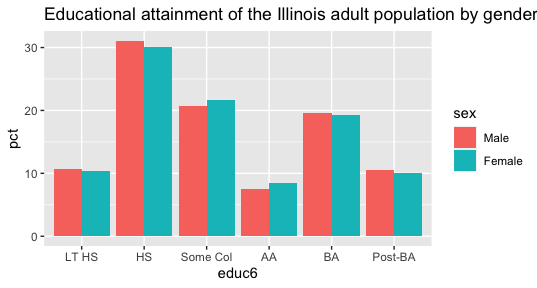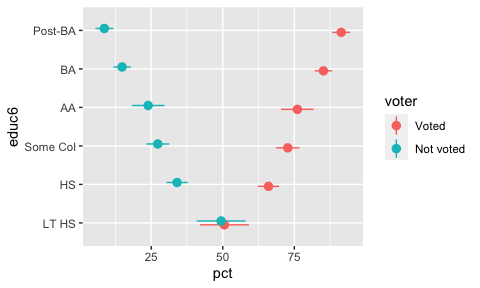crosstabs

library(pollster)
library(dplyr)
library(knitr)
library(ggplot2)

Crosstabs can come in wide or long format. Each is useful, depending on your purpose. Wide data is best for display tables. Long data is usually better for making plots, for instance..

Here is a wide table.

crosstab(df = illinois, x = sex, y = educ6, weight = weight) %>%
kable()
sex LT HS HS Some Col AA BA Post-BA n
Male 10.61114 31.08939 20.66614 7.498318 19.58311 10.55190 49108796
Female 10.37899 30.13231 21.64697 8.526414 19.26636 10.04895 53569718

And here is long format.

crosstab(df = illinois, x = sex, y = educ6, weight = weight, format = "long")
#> # A tibble: 12 x 4
#>    sex    educ6      pct         n
#>    <fct>  <fct>    <dbl>     <dbl>
#>  1 Male   LT HS    10.6  49108796.
#>  2 Male   HS       31.1  49108796.
#>  3 Male   Some Col 20.7  49108796.
#>  4 Male   AA        7.50 49108796.
#>  5 Male   BA       19.6  49108796.
#>  6 Male   Post-BA  10.6  49108796.
#>  7 Female LT HS    10.4  53569718.
#>  8 Female HS       30.1  53569718.
#>  9 Female Some Col 21.6  53569718.
#> 10 Female AA        8.53 53569718.
#> 11 Female BA       19.3  53569718.
#> 12 Female Post-BA  10.0  53569718.

By default, row percentages are used. You can also explicitly choose cell or column percentages using the pct_type argument. I discourage the use of column percentages–it’s better to just flip the x and y variables and make row percents–but the option is included to match functionality provided by other standard statistical software.

# cell percentages
crosstab(df = illinois, x = sex, y = educ6, weight = weight, pct_type = "cell")
#> # A tibble: 2 x 8
#>   sex    LT HS    HS Some Col    AA    BA Post-BA          n
#>   <fct>    <dbl> <dbl>      <dbl> <dbl> <dbl>     <dbl>      <dbl>
#> 1 Male      5.08  14.9       9.88  3.59  9.37      5.05 102678514.
#> 2 Female    5.41  15.7      11.3   4.45 10.1       5.24 102678514.

# column percentages
crosstab(df = illinois, x = sex, y = educ6, weight = weight, pct_type = "column")
#> # A tibble: 3 x 7
#>   sex       LT HS         HS Some Col        AA         BA  Post-BA
#>   <chr>       <dbl>      <dbl>      <dbl>     <dbl>      <dbl>      <dbl>
#> 1 Male         48.4       48.6       46.7      44.6       48.2       49.0
#> 2 Female       51.6       51.4       53.3      55.4       51.8       51.0
#> 3 n      10770999.  31409418.  21745113.  8249909.  19937965.  10565110.

To make a graph, just feed your tibble output to a ggplot2 function.

crosstab(df = illinois, x = sex, y = educ6, weight = weight, format = "long") %>%
ggplot(aes(x = educ6, y = pct, fill = sex)) +
geom_bar(stat = "identity", position = position_dodge()) +
labs(title = "Educational attainment of the Illinois adult population by gender")Margin of error

How the margin of error is calculated

The margin of error is calculated including the design effect of the sample weights, using the following formula:

sqrt(design effect)*zscore*sqrt((pct*(1-pct))/(n-1))*100

The design effect is calculated using the formula length(weights)*sum(weights^2)/(sum(weights)^2).

Get at topline table with the margin of error in a separate column using the moe_crosstab function. By default, a z-score of 1.96 (95% confidence interval is used). Supply your own desired z-score using the zscore argument. Only row and cell percents are supported. By default, the table format is long because I anticipate making visualizations will be the most common use-case for this graphic.

A wide format table looks like this.

ggplot2 offers multiple ways to visualize the margin of error. Here is one good option. (Please note, if you don’t have ggplot2 >= 3.3.0 you’ll get an error message.)Special case, the x-variable identifies survey waves

If the x-variable in your crosstab uniquely identifies survey waves for which the weights were independently generated, it is best practice to calculate the design effect independently for each wave. moe_wave_crosstab does just that. All of the arguments remain the same as in moe_crosstab.